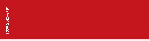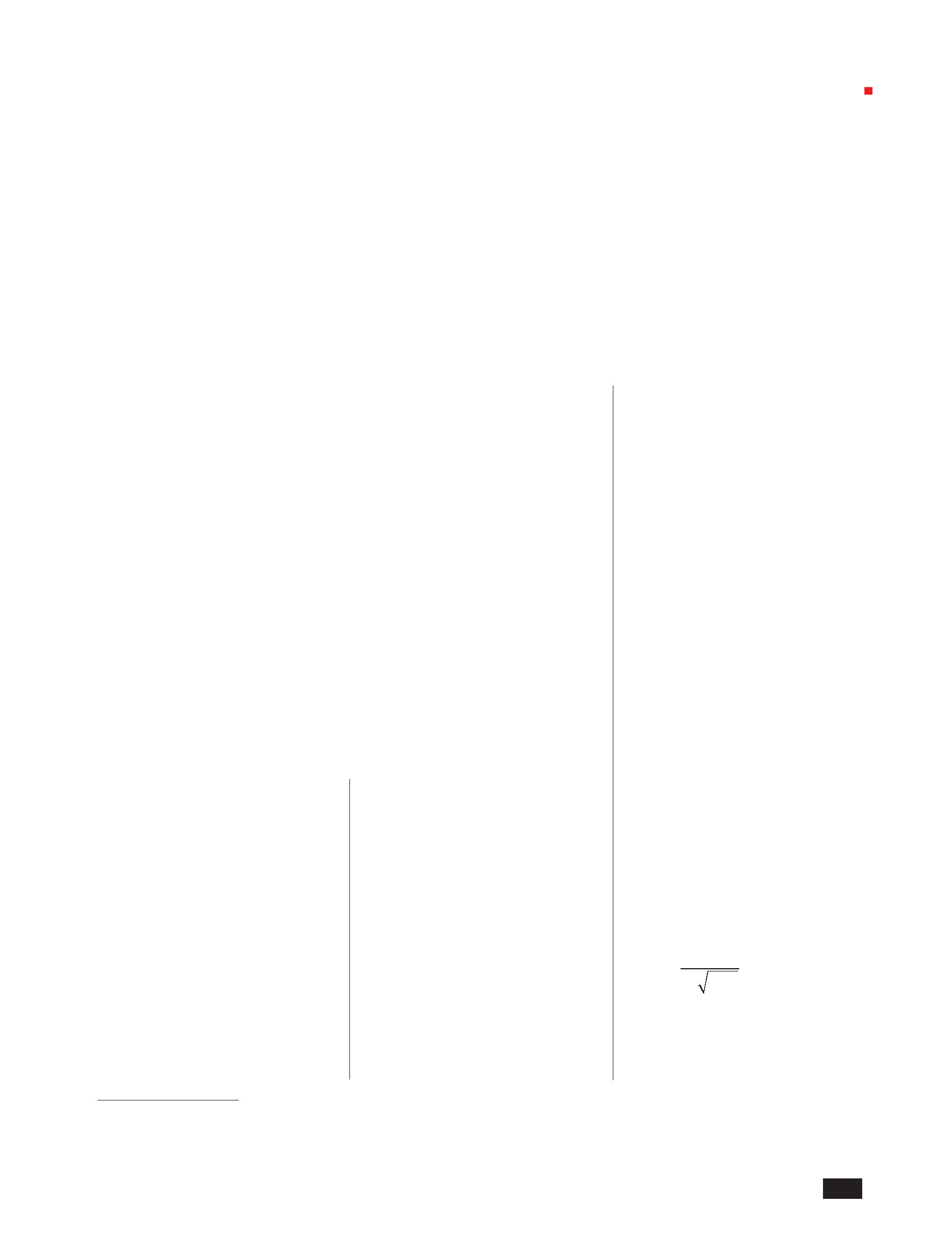=
f
m
1
Physical sciences | Physics
The effect of the dielectric layer thickness
on the negative permeability in metamaterials
Thi Trang Pham1,2, Ba Tuan Tong1,2, Thi Giang Trinh1, Hoang Tung Nguyen1, Minh Tuan Dang3, Dinh Lam Vu1*
1Institute of Materials Science, Vietnam Academy of Science and Technology (VAST)
2Hanoi University of Mining and Geology (HUMG)
3Hanoi - Amsterdam High School for the gifted
Received 20 April 2017; accepted 18 August 2017
Abstract:
the
thickness
of
the
dielectric
layer
In this work, we investigate the influences of the dielectric layer on the
has
been
concluded
to
be
extremely
magnetic resonance of the cut-wire pair structure (CWP). The interaction
significant.
However,
no
systematic
between the cut-wires is modeled through a LC circuit, based on which, the
magnetic resonant frequency is calculated. Furthermore, the dependence of
the resonant bandwidth on the structure parameters is also determined. By
tuning the dielectric layer thickness, we obtained a noticeable broadening of
the negative permeability regime of 17%, which represents the enhancement
of the magnetic resonance. A good agreement between the theory, simulation,
and practical experiment has been demonstrated. We believe that our results
should be consequential with regard to the determination of the mechanism
behind the wave-matter interaction in the GHz frequency regime.
Keywords: dielectric layer thickness, metamaterials, negative permeability
study on the influences of the dielectric
layer thickness currently exists.
In this work, we report the effects
of dielectric layer thickness on the
negative permeability bandwidth of
the conventional cut-wire pair (CWP)
structure from 12 to 18 GHz. The
negative permeability region is observed
to be broadened as a result of the
increased dielectric layer thickness. The
phenomenon is interpreted theoretically
by calculations on the LC circuit
Classification number: 2.1
model
and
verified
by
simulations
and
experiments
with
considerable
consistency.
Theoretical model
The
CWP
structure
includes
two
Introduction
In recent years, the revolution in
science and technology with regard
to seeking novel materials has gained
tremendous popularity throughout the
world. Metamaterials (MMs) have been
one of the most prominent candidates
in this regard due to their extraordinary
properties. Numerous potential
applications of MMs have been proposed
and demonstrated, such as biological
sensor , superlens , hi-low pass
filtering , antennas , invisible
[7-9]. Whereas, the permeability and
permittivity of MMs are simultaneously
negative in a common frequency
regime [10-13]. This unique property
of MMs is known to be arbitrarily
tuned in terms of the arrangement or
design of their compositions. In fact,
the negative permittivity region can be
obtained on a wide scale through the
periodic continuous-wire structure ,
but the negative permeability region is
restricted due to the resonant conditions.
Therefore, the realistic applications of
metal patterns on two sides of the
dielectric layer (Fig. 1A). Due to Zhou, et
al.’s great work , the CWP structure
can be modeled by an equivalent LC
circuit (Fig. 1B) by considering the CWs
as the inductors and the spaces between
the ends of the CW as the capacitors.
Furthermore, the resonant frequency
can be theoretically predicted as shown
below:
c (1)
l 2 c
cloaking
,
and
wireless
power
MMs are limited by the narrow negative
Since Eq. 1, the resonant frequency
transfer . Most of these applications
permeability bandwidth. From the large
depends
on
the
CW
length
and
the
are based on the unique optical property
amount of efforts made to expand the
dielectric constant of the middle layer.
of negative refractive index in MMs
negative permeability of MMs [15-17],
However,
some
experimental
results
*Corresponding author: Email:lamvd@ims.vast.vn
December 2017 Vol.59 Number 4
Vietnam Journal of Science,
Technology and Engineering
3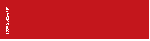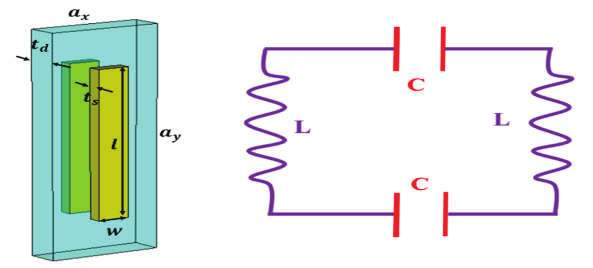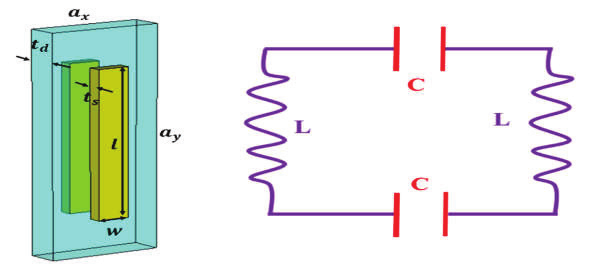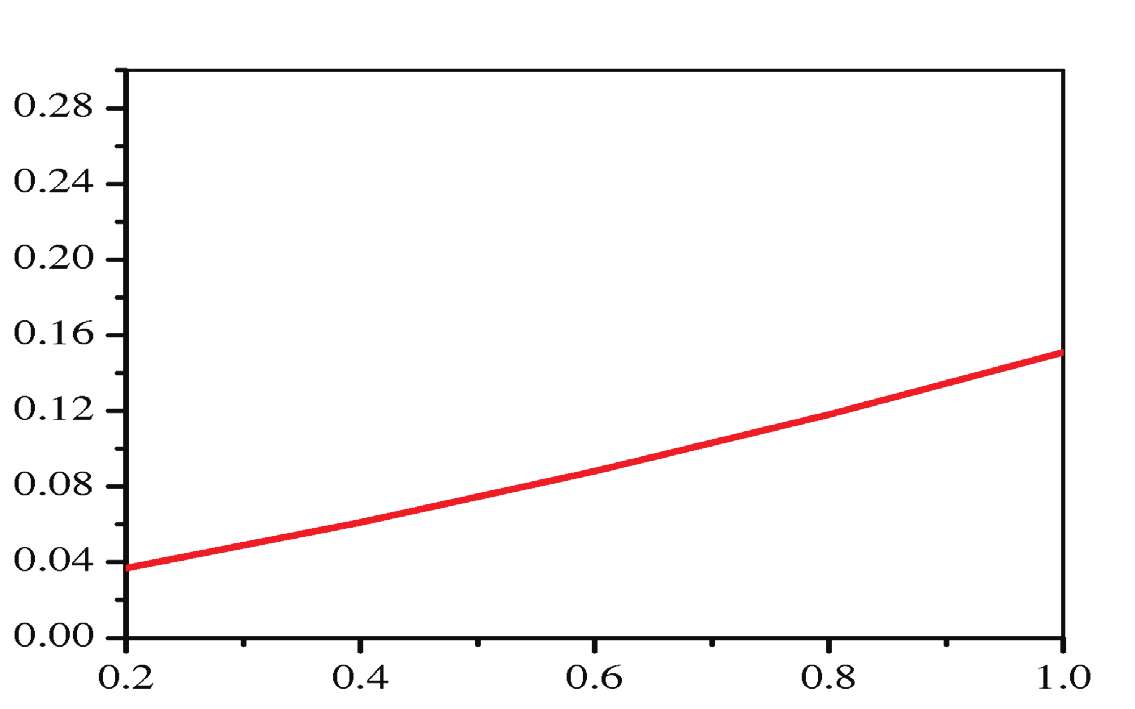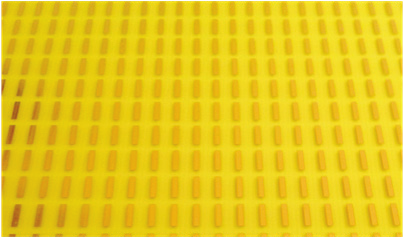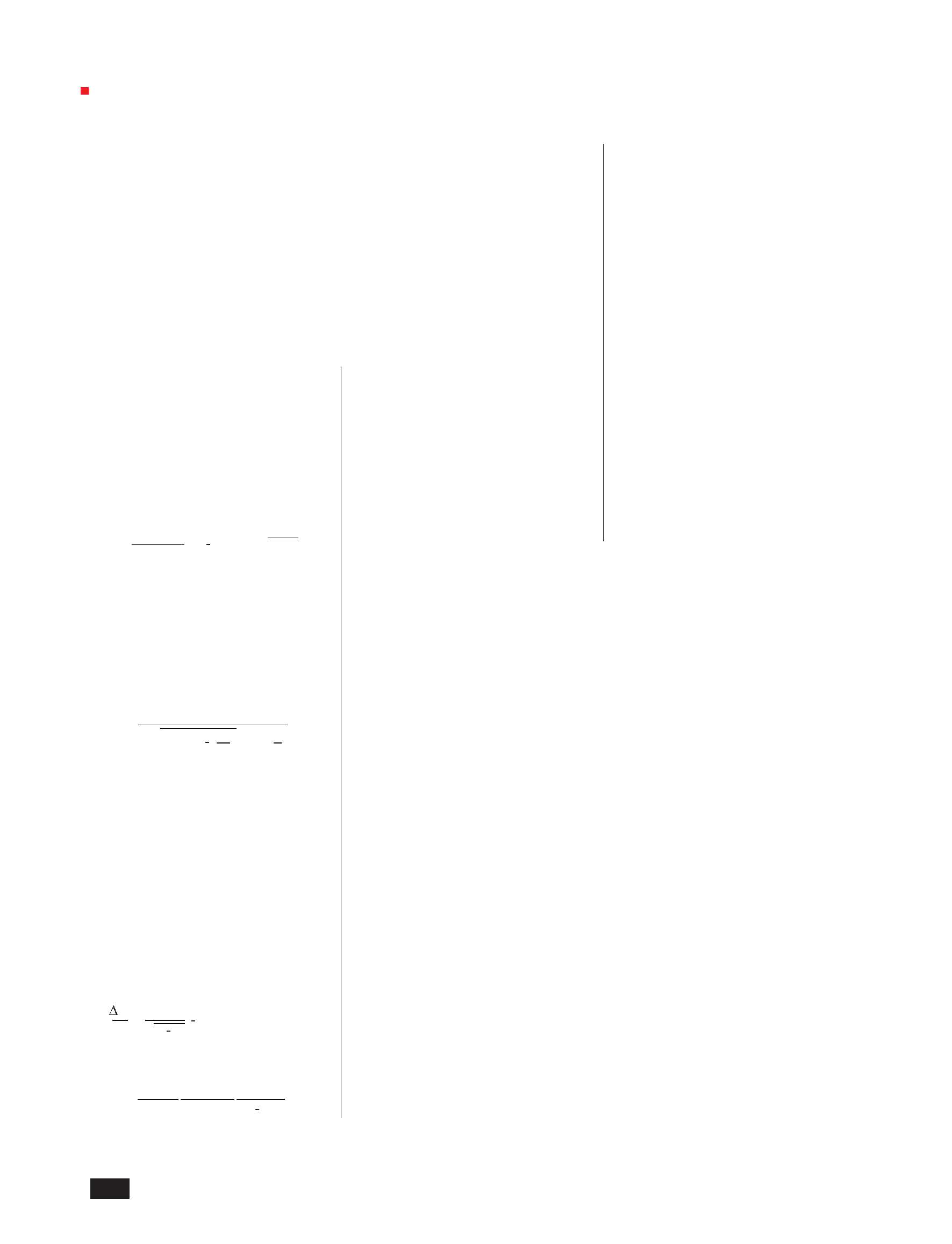� ��
=
0 1
C
d
0 d
s
d
1 1
� �
=
� =
Physical sciences | Physics
Simulation and experiment
The
proposed
CWP
structure
is
composed
of
a
dielectric
layer
FR4
with the dielectric constant as 4.3 and
copper patterns with ts = 0.036 mm, l
= 5.5 mm, w = 1 mm. The structure is
periodic along the x and y axis; the
(A) (B)
Fig. 1. (A) Unit cell and (B) the corresponding LC circuit of the CWP structure.
lattice constant for each direction is ax
= 3.6 mm and ay = 7.2 mm respectively.
The thickness of the dielectric layer is
reveal that dielectric thickness also
influences the resonant frequency [19,
20]. In our study, we propose a new
equation to calculate the inductance
and capacitance in the equivalent
LC circuit, in which the dielectric
thickness is considered according to the
hybridization model:
clw
� = 2(� + �)(�2�) td (2)
Since t is considered to fall in the
range between 0.2 to 1.0 mm, F is
always greater than 0 and smaller than 1.
Hence, Eq. 4 is positive and identifiable.
The relation between the dielectric layer
thickness and the negative permeability
bandwidth can be realized in Eq. 4 and
Eq. 5 or more clearly in Fig. 2, where
the evolution of Δf/f according to t is
presented.
tuned from 0.2 mm to 1.0 mm by a step
of 0.2 mm. The samples are prepared
on the standard printed circuit boards
(PCB) through the application of the
conventional photolithography method
(Fig. 3). The simulations are operated
on the simulation program CST ,
and the measurements are performed by
the vector network analyzer system to
obtain the scattering parameters.
where: l is the length, w constitutes the
width, and t forms the thickness of a
CW; t represents the dielectric layer
thickness and c is a constant 0.2 c
0.3.
Hence, the resonant frequency of the
equivalent LC circuit becomes.
� =
���2�� �1 ���/(1 + )
(3)
In Eq. 3, the resonant frequency is
shown to depend on the the width of the
CW and the dielectric layer thickness.
It is worth noting that in the case where
(ts w) and (ts td), Eq. 3 assumes the
same form as Eq. 1 of J. Zhou, et al. .
td (mm)
Fig. 2. The dependence of the negative permeability fractional bandwidth on
the dielectric layer thickness presented by theoretical model.
In
other
words,
the
mutual
coupling
effect between the CWs has not been
included in the calculations of J. Zhou,
et
al.
.
Therefore,
the
fractional
bandwidth of the negative permeability
can be expressed as follows:
� 1
√1 �
1
(4)
where: F is defined as:
� (� + �)
� �2�
(5)
Fig. 3. Fabricated sample with the presented structure parameters.
4
Vietnam Journal of Science,
Technology and Engineering
December 2017 Vol.59 Number 4
Δf/f0